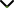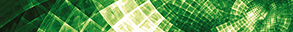# Topological Indices, and Applications of Graph Theory

Publishing date
01 Aug 2021
Status
Closed
26 Mar 2021

Guest Editors

1Bursa Uludag University, Bursa, Turkey

2Sungkyunkwan University, Suwon, Republic of Korea

3Selcuk University, Konya, Turkey

This issue is now closed for submissions.

# Topological Indices, and Applications of Graph Theory

This issue is now closed for submissions.

## DescriptionA topological graph index, also called a molecular descriptor, is a mathematical formula that can be applied to any graph which models some molecular structure. From this index, it is possible to analyse mathematical values and further investigate some physicochemical properties of a molecule. Therefore, it is an efficient method in avoiding expensive and time-consuming laboratory experiments.

Molecular descriptors play a significant role in mathematical chemistry, especially in quantitative structure-property relationship (QSPR), and quantitative structure-activity relationship (QSAR) investigations. An example of a molecular descriptor is a topological descriptor. Nowadays, there are numerous topological indices, some applied in chemistry. They can be classified by the structural properties of the graphs used for their calculation. For instance, there is the Hosoya index, calculated by counting non-incident edges in a graph. Furthermore, the Estrada index is based on the spectrum of the graph, the Randić connectivity index, and the Zagreb group indices are calculated using the degrees of vertices, etc. Perhaps the best known, and most widely used topological indice is the Wiener index which is based on the topological distance of vertices in the respective graph. It was defined and used by Harold Wiener in 1947, which helped him to compare the boiling points of some alkane isomers. Since then, over 3000 topological graph indices are registered in Chemical Data Bases. This research area is studied by mathematicians and chemists. There is a rapidly increasing interest in this topic, therefore topological graph indices are researched worldwide.

The aim of this Special Issue is to solicit original research articles focussing on topological graph indices, and applications of graph theory. We highly encourage submissions from researchers in the field of mathematical chemistry. Review articles discussing the state of the art are also welcome.

Potential topics include but are not limited to the following:

• Molecular graphs
• Topological graph indices
• Chemical applications of graph indices
• Mathematical properties of graph indices
• Energy of graphs
• Spectral graph theory
• Graph matrices
• Graph parameters
• Derived graphs
• Applications of graphs
• Combinatorics
• Labelling in graphs
• Graph polynomials
• Chromatic graph theory
• Matching in graphsJournal metrics
See full report
Acceptance rate24%
Submission to final decision69 days
Acceptance to publication35 days
CiteScore1.500
Journal Citation Indicator1.140
Impact Factor1.4Author guidelinesEditorial boardDatabases and indexing

Article of the Year Award: Impactful research contributions of 2022, as selected by our Chief Editors. Discover the winning articles.